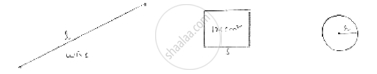Share

# A Steel Wire When Bent is the Form of Square Encloses an Area of 12 Cm2. If the Same Wire is Bent in Form of Circle. Find the Area of Circle. - CBSE Class 10 - Mathematics

ConceptPerimeter and Area of a Circle

#### Question

A steel wire when bent is the form of square encloses an area of 12 cm2. If the same wire is bent in form of circle. Find the area of circle.

#### SolutionLet side of square = s and length of wire be l. As wire is bent into square

l = perimeter of square = 4s.

Area of square = 121cm2 = s2.

S = sqrt(121) = 11𝑐𝑚

∴ length of wire l = 4(11) = 44cm

As wire is bent into circle (let radius be r)

Length of wire = circumference

44 = 2𝜋r

22/7 × 2 × r= 44 ⇒ r = (44×7)/(2×22) = 7𝑐𝑚
𝐴𝑟𝑒𝑎 𝑜𝑓 𝑐𝑖𝑟𝑐𝑙𝑒 = 𝜋𝑟2

= 22/7 × 7 × 7

= 22 × 7

= 154𝑐𝑚2

Is there an error in this question or solution?

#### Video TutorialsVIEW ALL 

Solution A Steel Wire When Bent is the Form of Square Encloses an Area of 12 Cm2. If the Same Wire is Bent in Form of Circle. Find the Area of Circle. Concept: Perimeter and Area of a Circle.
S